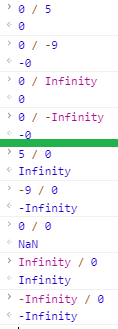#2018 iT 邦幫忙鐵人賽DAY 6
9
Modern Web

## 運算式 (Expression) 與 運算子(Operator)

JavaScript 的語法基本上可以分為兩大類，「敘述句 (Statement)」「運算式 (Expression)」

• 敘述句 (Statement)：
簡單來說，敘述句就是執行某個動作。 像是變數的宣告、賦值，迴圈和 `if` 判斷式等等都可以被歸類於此。
``````var foo;
``````
• 運算式 (Expression)：
而運算式最大的特性，就是它會產生一個「值」。
像是我們在呼叫 `function` 時的參數 (arguments)，或者透過 `=` 賦值時，在 `=` 「右側」的部分都屬於運算式的部分。
``````var a = 10 * 10;
``````

• 算術運算子 (Arithmetic Operator)
• 指派運算子 (Assignment Operator)
• 位元運算子 (Bitwise Operator)
• 比較運算子 (Comparison Operator)
• 邏輯運算子 (Logical Operator)
• 字串運算子 (String Operator)
• 特殊運算子 (Special Operator)

## 算術運算子

JavaScript 各種運算子當中，最常見的就屬「算術運算子」了。

### 加號 (+)

``````var a = 1 + 2;
console.log(a);      // 3
``````

`Infinity``-Infinity`，以及 `NaN` 都屬於「特殊的數字」。

``````Infinity + Infinity      // Infinity

-Infinity + -Infinity    // -Infinity

-Infinity + Infinity     // NaN
``````

`NaN` 的話，則是只要有其中一個是 `NaN`，那麼結果就必定是 `NaN`

``````10 + NaN            // NaN

Infinity + NaN      // NaN

-Infinity + NaN     // NaN
``````

``````100 + "100"      // "100100"

100 + "ABC"      // "100ABC"

"ABC" + "XYZ"    // "ABCXYZ"
``````

`number``boolean``object` 的情況來說，轉型時會去呼叫它們的 `.toString()` 的 「原型方法」[註1] 去取得對應的字串。
`null``undefined` 則是透過 JavaScript 的 `String()` 函數來將它們分別轉為 `"null"``"undefined"`

``````10 + 'abc'          // "10abc"
true + 'abc'        // "trueabc"

100 + {}            // "100[object Object]"

// 當數字與 undefined 相加時，undefined 會被嘗試轉成數字，也就是 NaN
123 + undefined     // NaN
"abc" + undefined   // "abcundefined"

// 當數字與 null 相加時，null 會被嘗試轉成數字，也就是 0
123 + null          // 123
"123" + null        // "123null"
``````

``````var num1 = 10;
var num2 = 100;

var str = "10 加 100 的數字會是" + num1 + num2;
``````

``````var num1 = 10;
var num2 = 100;

var str = "10 加 100 的數字會是" + (num1 + num2);
``````

`str` 的結果就會是預期中的「"10 加 100 的數字會是110"」了。

### 減號 (-)

``````var a = 10 - 3 ;
console.log(a);      // 7
``````

``````Infinity - Infinity      // NaN
-Infinity - -Infinity    // NaN

-Infinity - Infinity     // -Infinity
Infinity - -Infinity     // Infinity
``````

• 基本型別 (如 `string``boolean``undefined``null`)
在做減法運算時，若其中一方屬於基本型別且不是數字的情況，那麼 JavaScript 會在先在背後透過 `Number()` 嘗試將數值轉為「數字」，再進行運算。
``````100 - "50"        // 50
100 - "abc"       // NaN

// false 經過 Number() 轉型成數字後，會變成 0
100 - false       // 100
// true 經過 Number() 轉型成數字後，會變成 1
100 - true        // 99

100 - undefined   // NaN
100 - null        // 100
``````
• 物件型別
如果是物件型別的情況下，則是會先透過物件的 `valueOf()` 方法 [註2] 先求得對應的數值，如果得到 `NaN`，那麼結果就是 `NaN`
如果物件沒有 `valueOf()` 方法的話，則是透過 `toString()` 先轉成「字串」後，再以 `Number()` 嘗試將數值轉為「數字」後進行運算。
``````// 簡單物件
100 - { }         // NaN
``````
``````// 自訂物件，透過 Object.prototype.valueOf 來指定物件的 value

function Obj(number) {
this.num = number;
};

Obj.prototype.valueOf = function(){
return this.num;
};

var o = new Obj(50);

// 因為 o.valueOf() 的值為 50
100 - o         // 50
``````

### 乘號 (*)

``````var a = 10 * 10 ;
console.log(a);      // 100
``````

``````100 * "10"      // 1000
100 * abc       // NaN

100 * true      // 100
100 * false     // 0

100 * {}        // NaN
``````

### 除號 (/)

``````var a = 100 / 10 ;
console.log(a);      // 10
``````

• 被除數為正數，則結果為 `Infinity`
• 被除數為負數，則結果為 `-Infinity`
• 被除數為 `0`，則結果為 `NaN`

### 取餘數 (%)

``````var a = 100 % 33;
console.log(a);      // 1
``````

``````Infinity % 0              // NaN
Infinity % 100            // NaN
Infinity % Infinity       // NaN
Infinity % -Infinity      // NaN
``````

``````100 % Infinity       // 100
0 % Infinity         // 0
``````

• [註1] 原型方法: JavaScript 與其他物件導向語言不一樣的地方是，它的繼承是 "prototype-base" 的。 也就是說，即便是基本型別的數值，除了 `null``undefined` 屬於特殊用途，並沒有相對應的原始型別包裹物件之外，都有它們對應的構造函數，或稱包裝器 (wrapper) 。 而這些「基本型別」的數值理論上是不會有對應的方法 (method)，但可以由原始物件繼承而來。這部分在後續講到原型鍊時會有詳細說明。

• [註2] `valueOf()` 是用來回傳特定物件相對應原始型別的值，當 JavaScript 的物件在進行運算時，都會透過 `valueOf()``toString()` 方法，取回該物件對應的原始型別的值再進行運算。

### 3 則留言

0Kuro Hsu iT邦新手 4 級 ‧ 2020-05-26 10:27:19 檢舉

0

Kuro Hsu iT邦新手 4 級 ‧ 2020-10-15 15:35:22 檢舉

`100 + {}` 的時候，JavaScript 會嘗試將 `{}` 透過 `toString()` 自動轉型，這裡你可以試試：

``````const obj = {};
obj.toString();  // "[object Object]"
``````

denny3299 iT邦新手 5 級 ‧ 2020-10-15 21:16:32 檢舉